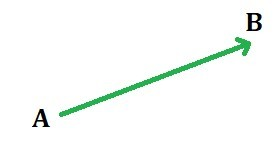# Vector Projection using Python

A vector is a geometric object which has both magnitude (i.e. length) and direction. A vector is generally represented by a line segment with a certain direction connecting the initial point A and the terminal point B as shown in the figure below and is denoted by## Projection of a Vector on another vector

The projection of a vectoronto another vectoris given asComputing vector projection onto another vector in Python:

 # import numpy to perform operations on vector  import numpy as np     u = np.array([1, 2, 3])   # vector u  v = np.array([5, 6, 2])   # vector v:     # Task: Project vector u on vector v     # finding norm of the vector v  v_norm = np.sqrt(sum(v**2))         # Apply the formula as mentioned above  # for projecting a vector onto another vector  # find dot product using np.dot()  proj_of_u_on_v = (np.dot(u, v)/v_norm**2)*v     print("Projection of Vector u on Vector v is: ", proj_of_u_on_v)

Output:

Projection of Vector u on Vector v is:  [1.76923077 2.12307692 0.70769231]

One liner code for projecting a vector onto another vector:

 (np.dot(u, v)/np.dot(v, v))*v

## Projection of a Vector onto a Plane

The projection of a vectoronto a plane is calculated by subtracting the component ofwhich is orthogonal to the plane from.where,is the plane normal vector.

Computing vector projection onto a Plane in Python:

 # import numpy to perform operations on vector  import numpy as np     # vector u   u = np.array([2, 5, 8])            # vector n: n is orthogonal vector to Plane P  n = np.array([1, 1, 7])             # Task: Project vector u on Plane P     # finding norm of the vector n   n_norm = np.sqrt(sum(n**2))          # Apply the formula as mentioned above  # for projecting a vector onto the orthogonal vector n  # find dot product using np.dot()  proj_of_u_on_n = (np.dot(u, n)/n_norm**2)*n     # subtract proj_of_u_on_n from u:   # this is the projection of u on Plane P  print("Projection of Vector u on Plane P is: ", u - proj_of_u_on_n)

Output:

Projection of Vector u on Plane P is:  [ 0.76470588  3.76470588 -0.64705882]

My Personal Notes arrow_drop_upCheck out this Author's contributed articles.

If you like GeeksforGeeks and would like to contribute, you can also write an article using contribute.geeksforgeeks.org or mail your article to contribute@geeksforgeeks.org. See your article appearing on the GeeksforGeeks main page and help other Geeks.

Please Improve this article if you find anything incorrect by clicking on the "Improve Article" button below.

Article Tags :
Practice Tags :

1

Please write to us at contribute@geeksforgeeks.org to report any issue with the above content.